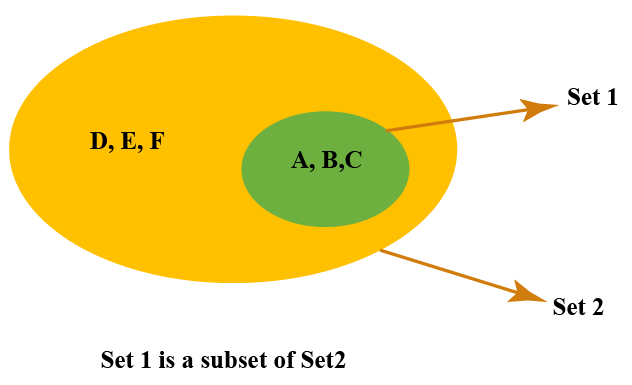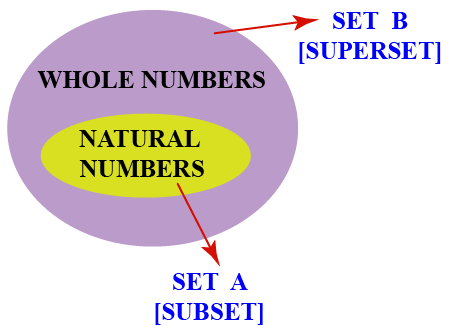# Subset

Subset

A set is a well-defined collection of numbers, alphabets, objects, or any items.

A subset is a part of the set.

If \begin{align} Set\:1 = \left \{ {A,B,C} \right \}\end{align} and \begin{align} Set\:2 = \left \{ {A,B,C,D,E,F} \right \}\end{align} we can say that \begin{align} Set \:1 \end{align} is a subset of \begin{align} Set \:2 \end{align} since all the elements in set 1 are present in set 2In this lesson, you will learn about subsets, subset symbols, types of subsets, and their properties. You will also learn the differences between proper subset and improper subset. You will go through some solved examples and also be able to solve some interesting practice questions toward the end of the lesson.

## Lesson Plan

 1 What is a Subset? 2 Important Notes 3 Challenging Questions 4 Solved Examples on Subset 5 Interactive Questions on Subset

## What Is a Subset?

A set is a collection of different objects.

Set A is a subset of set B if all the elements in set A are present in set B.

Here, A is called the subset, and B is called the superset.

For example, let \begin{align} Set\: A \:=\: \{{All\: whole\: numbers} \}\end{align}, \begin{align} Set\: B \:=\:\{ {All\: natural\: numbers}\} \end{align}. We can say that set A is the super set of set B.## What Is the Symbol of a Subset?

• The symbol for subset is '\begin{align} \subseteq\end{align}'
• It is read as "is a subset of."

For example, if \begin{align} Set A = \left \{ {1,2,3} \right \}\end{align} and \begin{align} Set B = \left \{ {1,2,3,4,5,6} \right \}\end{align} we can say:

 \begin{align}A\sqsubseteq B\end{align} (read as A is a subset of B)

## What Are the Types of Subsets?

There are two types of subsets.

• Proper subsets
• Improper subsets

### Proper Subset

• A subset is said to be a proper subset if at least one of the elements in set A is present in set B
• It is denoted by the symbol "\begin{align} \subset \end{align}"
• The formula to calculate number of proper subsets is \begin{align} 2^n -1 \end{align} (where n is the number of elements in the set)

Example: \begin{align} A = \left \{ {10,20} \right \}\end{align}

Total number of elements \begin{align}(n) = 2 \end{align}

Number of proper subsets  = \begin{align}2^2-1 \end{align}

= \begin{align}4-1 \end{align}

= \begin{align}3 \end{align}

Therefore, there are 3 elements in the proper subset which are \begin{align}\left \{ \left \{ \right \},\left \{ 10 \right \},\left \{ 20 \right \} \right \}\end{align}

### Improper Subset

• A subset that has every element of the other set is called an improper subset.
• It is denoted by the symbol "\begin{align} \sqsubseteq \end{align}"

Example: Set X = \begin{align}\left \{ 5,10,15 \right \}\end{align}

The subsets of set X are:

$$\left \{ {} \right \}$$,$$\left \{ {5} \right \}$$,$$\left \{ {10} \right \}$$,$$\left \{ {15} \right \}$$,$$\left \{ {5,10} \right \}$$,$$\left \{ {5,15} \right \}$$,$$\left \{ {10,15} \right \}$$,$$\left \{ {5,10,15} \right \}$$

Here the subset {5,10,15} has all the elements of the original set and therefore we can say

\begin{align}\left \{ {5,10,15} \right \} \sqsubseteq X \end{align}Challenging Questions
1. Set A = List of all prime numbers from 1 to 10
Set B = All the numbers from 1 to 10
List out all the subsets and proper subsets.
2. Set P = {'S', 'U', 'B', 'S', 'E', 'T'}. Find the number of subsets.

## What is a Power Set?

• A power set is a collection of all the subsets together along with the empty set and the original set.
• It is denoted by the notation P(A) for a set A.
• Given there are $$n$$ number of elements in a set, then there will be exactly 2elements in the power set.

Example

Let us understand this with an example.

Set \begin{align} X = \left \{ {2,4,6} \right \}\end{align}

Total number of elements in the set \begin{align} X =3\end{align}

The power set of X is

$$P( X )=$$ $$\left \{ \right \}$$,$$\left \{ 2 \right \}$$,$$\left \{ 4 \right \}$$,$$\left \{ 6 \right \}$$,$$\left \{ 2,4 \right \}$$,$$\left \{ 4,6 \right \}$$,$$\left \{ 2,6 \right \}$$,$$\left \{ 2,4,6\right \}$$

\begin{align} P( X )= 2^n\end{align}

Substituting \begin{align} n\:=\:3 \end{align}

\begin{align} P( X )= 2^3\end{align}

## What Are the Properties of Subsets?

• Any given set is a subset of itself. If \begin{align} Set \:A = \left \{ {1,2,3} \right \}\end{align}, we can say that set A is always a subset of set A, which means A \begin{align} \subset\end{align} A.
• An empty set is a subset of every set.
• If A is a subset of B, then we can say that all the elements in A are present in B.
• The set which has all its elements in the other set is called a subset and the original set is called a superset.
• There are \begin{align} 2^n \end{align} subsets and \begin{align} 2^n - 1 \end{align} proper subsets for a given set.

## 1. Is an empty set a subset?

Yes, an empty set is a subset of every set since there are no elements in an empty set and it is a part of every set.

## 2. Is Phi a proper subset?

Yes, Phi is a proper subset. It is included every time when we write elements of a proper subset.

## 3. Is 0 an element of an empty set?

An empty set does not have any values. Hence, 0 is not an element of an empty set.

More Important Topics
Numbers
Algebra
Geometry
Measurement
Money
Data
Trigonometry
Calculus
More Important Topics
Numbers
Algebra
Geometry
Measurement
Money
Data
Trigonometry
Calculus